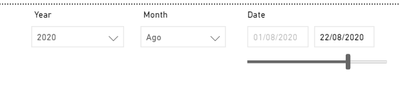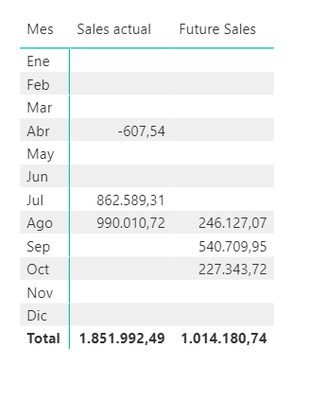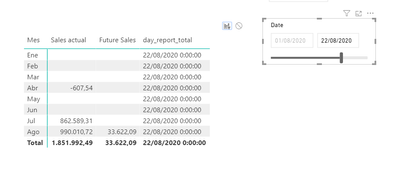cancel
Showing results for
Did you mean:Frequent Visitor

## I need to create a date to use as VAR

Hello community,

I need to create a measure that has a fixed variable, to perform the future calculation,

Here is the process I am following:

The filters I have are the following:I need to calculate two measures the sales you make to date and on the other hand the reserved sales that I have in the future, depending on the date selected in Date

This is the result I want to get:The measures used are the following:

``Sales actual = SUM(f_sales[base])``

``````Future Sales =
VAR FECHAINFORME = DATE(2020,08,22)
RETURN
CALCULATE(
,f_reserva[externa]= 0
, FILTER(
f_reserva
,f_reserva[fecha]>= FECHAINFORME
))``````

As you can see I am using the DATE () function, but what I want is for this date to be the maximum selected in the Date filter.

I have tried replacing DATE with the following measure:

``````day_report_total = CALCULATE(
MAX('Calendario'[Date])
,ALLSELECTED('Calendario')
)``````

But the result is the following:Thank you very much for your help

1 ACCEPTED SOLUTIONCommunity Champion

@arossello Attaching the file below my signature. I have added a disconnected slicer to filter the dates, Let me know if that is what you want.

``````Future Sales =
VAR DisMaxDate =
CALCULATE (
MAX ( 'Disconnected Calendario'[Date] ),
ALLSELECTED ( 'Disconnected Calendario'[Date] )
)
VAR VisualMaxDate =
MAX ( Calendario[Date] )
VAR Result =
SUMX (
FILTER ( VALUES ( Calendario[Date] ), Calendario[Date] > DisMaxDate ),
"@Sales", [Total Sales]
),
[@Sales]
)
RETURN
Result
``````
``````Current Sales =
VAR DisMaxDate =
CALCULATE (
MAX ( 'Disconnected Calendario'[Date] ),
ALLSELECTED ( 'Disconnected Calendario'[Date] )
)
VAR Result =
SUMX (
FILTER ( VALUES ( Calendario[Date] ), Calendario[Date] <= DisMaxDate ),
"@Sales", [Total Sales]
),
[@Sales]
)
RETURN
Result
``````

6 REPLIES 6Community Champion

@arossello Attaching the file below my signature. I have added a disconnected slicer to filter the dates, Let me know if that is what you want.

``````Future Sales =
VAR DisMaxDate =
CALCULATE (
MAX ( 'Disconnected Calendario'[Date] ),
ALLSELECTED ( 'Disconnected Calendario'[Date] )
)
VAR VisualMaxDate =
MAX ( Calendario[Date] )
VAR Result =
SUMX (
FILTER ( VALUES ( Calendario[Date] ), Calendario[Date] > DisMaxDate ),
"@Sales", [Total Sales]
),
[@Sales]
)
RETURN
Result
``````
``````Current Sales =
VAR DisMaxDate =
CALCULATE (
MAX ( 'Disconnected Calendario'[Date] ),
ALLSELECTED ( 'Disconnected Calendario'[Date] )
)
VAR Result =
SUMX (
FILTER ( VALUES ( Calendario[Date] ), Calendario[Date] <= DisMaxDate ),
"@Sales", [Total Sales]
),
[@Sales]
)
RETURN
Result
``````Frequent Visitor

@AntrikshSharma  Thank you very much, for the solution provided it is greatSuper User

@arossello , this should have worked. Try like this once

Future Sales =
VAR _FECHAINFORME = maxx(ALLSELECTED('Calendario'),'Calendario'[Date])
RETURN
CALCULATE(
,f_reserva[externa]= 0
, FILTER(
f_reserva
,f_reserva[fecha]>= _FECHAINFORME
))Frequent Visitor

I have had a problem if I apply your measure, the result is the following:The amount is that of 08/22/2020, as you can see future months do not appear.

I don't know what I can do anymore😥Super User

@arossello , try like

Future Sales =
VAR _FECHAINFORME = maxx(ALLSELECTED('Calendario'),'Calendario'[Date])
RETURN
CALCULATE(
,f_reserva[externa]= 0
, FILTER(
all(f_reserva)
,f_reserva[fecha]>= _FECHAINFORME
))

Can you share sample data and sample output in table format?Frequent Visitor

@amitchandak  , Hello

I am attaching the pbix, so you can see the behavior of the data.
The biggest problem is that when we select a date that is not the end of the month it works well.But if we select the last day of the month this is the result, as you can see the measure does not work